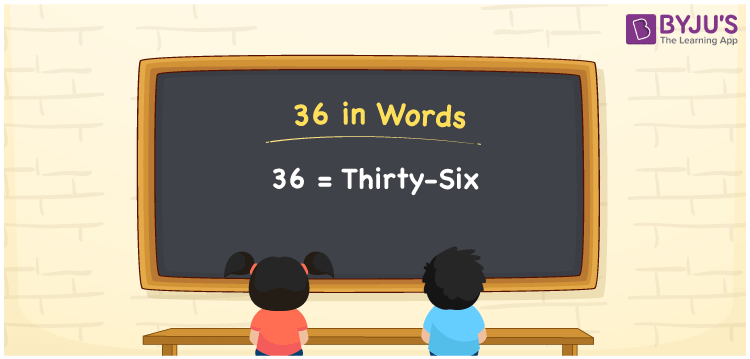# 36 in Words

36 in words is written as Thirty-six. In both the International and the Indian System of Numerals, 36 in words is written as Thirty-six. The number 36 is a cardinal number as 36 can represent some quantity. For example, “36 persons are waiting” here, 36 indicates the number of people.

 36 in words Thirty-six Thirty-six in numbers 36

## 36 in English Words

We write 36 in English Words using the letters of English alphabets. Therefore, we spell 36 in English as “Thirty-six.”## How to Write 36 in Words?

To write 36 in words, we shall use the place value chart. In the place value chart, write 6 in the ones place and 3 in the tens place. Let us make a place value chart to write the number 36 in words.

 Tens Ones 3 6

Thus, we can write the expanded form as

3 × Ten + 6 × One

= 3 × 10 + 6 × 1

= 30 + 6

= 36

= Thirty-six.

36 is the 36th natural number that is succeeded by 35 and preceded by 37.

36 in words – Thirty-six

• Is 36 an odd number? – No
• Is 36 an even number? – Yes
• Is 36 a perfect square number? – Yes; 6 × 6 = 36
• Is 36 a perfect cube number? – No
• Is 36 a prime number? – No
• Is 36 a composite number? – Yes

## Frequently Asked Questions on 36 in Words

Q1

### How to write 36 in English words?

36 in words is written as Thirty-six.
Q2

### How to spell 36?

The spelling of 36 is “Thirty-six.”
Q3

### Is 36 a Whole Number?

Yes, 36 is a Whole Number.